## How to create a formula field in salesforce

How to create a formula field in salesforce :- In this Salesforce Tutorial we are mainly going to concentrate about implementing business logic. So what is business logic and how we implement business logics in salesforce? In implement business logics in salesforce we have understand about Formula fields, Validation rules, Field Dependencies.

## What is a Formula Field in Salesforce?

Formula Field is a custom field generated by system of data type Formula. It is a read-only field which means we can not edit Formula Field.

• Formula Field in a read-only field.
• Formula Field derives the value from Formula expression.
• When the source field get changed automatically the Formula field changes(Updated).
• Formula in Salesforce are used to calculate custom fields, validation rules, Flow logic etc.
• Using Formula Editor we create formula in Salesforce.

### Formula Editor and it’s Features.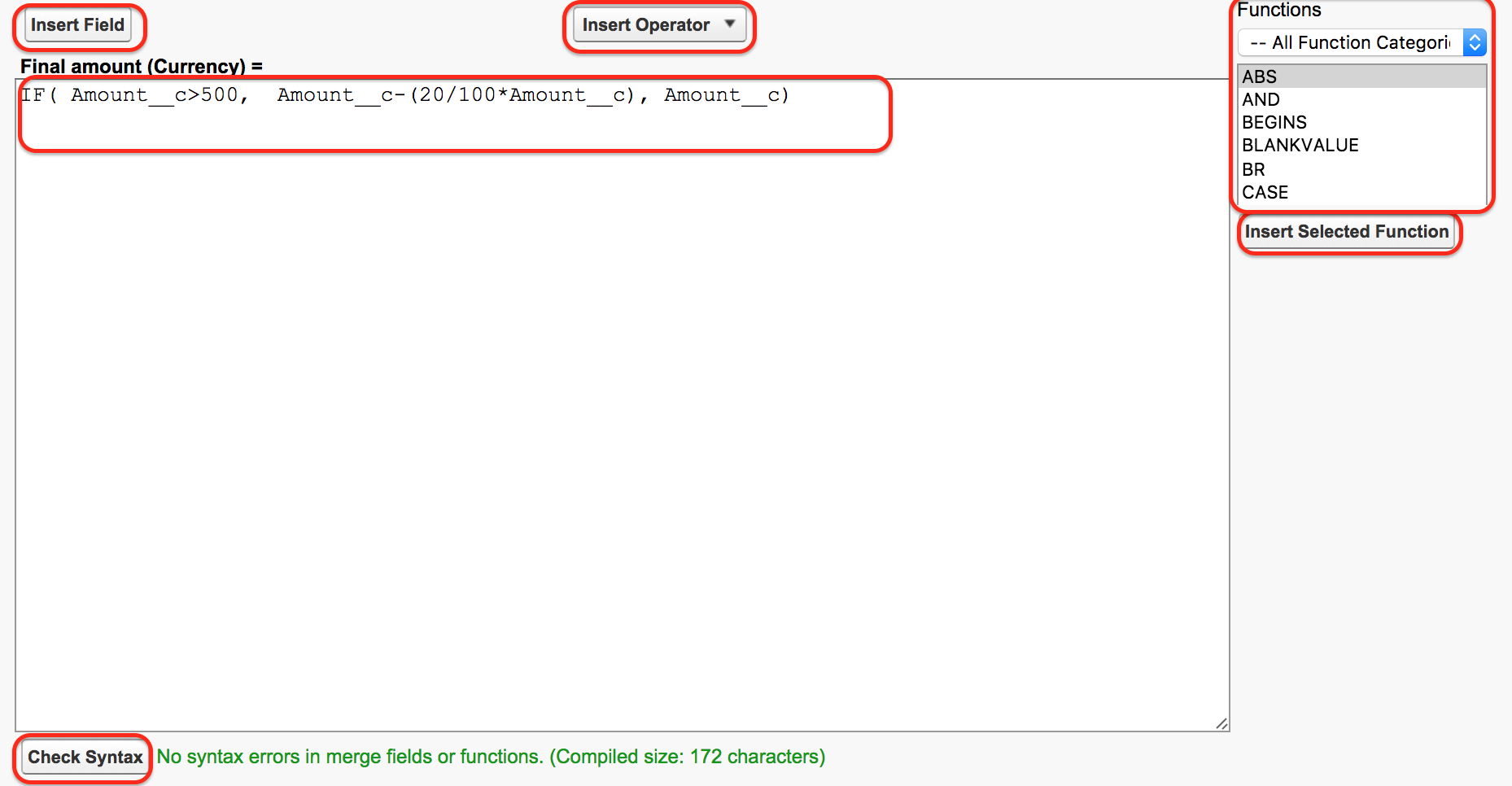In Salesforce Editor, we use different elements while creating various Formula fields in Salesforce. As shown above figure we have noted various elements. They are

1. Text Area :- It is the Space where we enter Formula.
2. Insert Field :- It allows user to select fields to use in our Formula.
3. Insert Operator:- Insert Operator display list of all Mathematical and Logical operators present in salesforce.
4. Functions Menu:- Using functions menu we insert formula functions like AND, ISNUMBER, CASE, NOT etc
5. Check Syntax :- To check the formula syntax is valid or not.

## How to create a formula field in salesforce.

In this Salesforce tutorial we are going to create a formula field for Invoice Object. Here we are going to create Formula for discount amount. Where the discount has to be applied for all purchase above 500\$.

Syntax : `If Invoice Amount > 500, final Amount = Invoice Amount - (20/100*Amount__c), Amount__c).`

As per above formula discount will be applied for only above 500\$ purchases only else Final amount will be the invoice amount. Let us create a formula field in salesforce.

## How to create a formula field in salesforce.

Go to Setup | Build | Create | Object | Invoice | Create new Filed.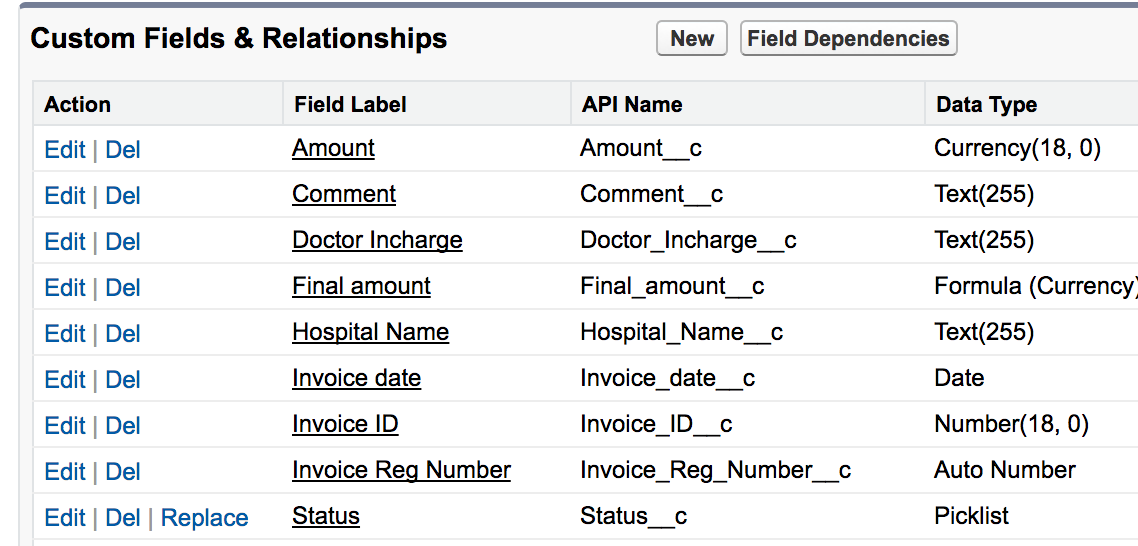Here select the object visit that you want to create Formula field and click on New Custom field.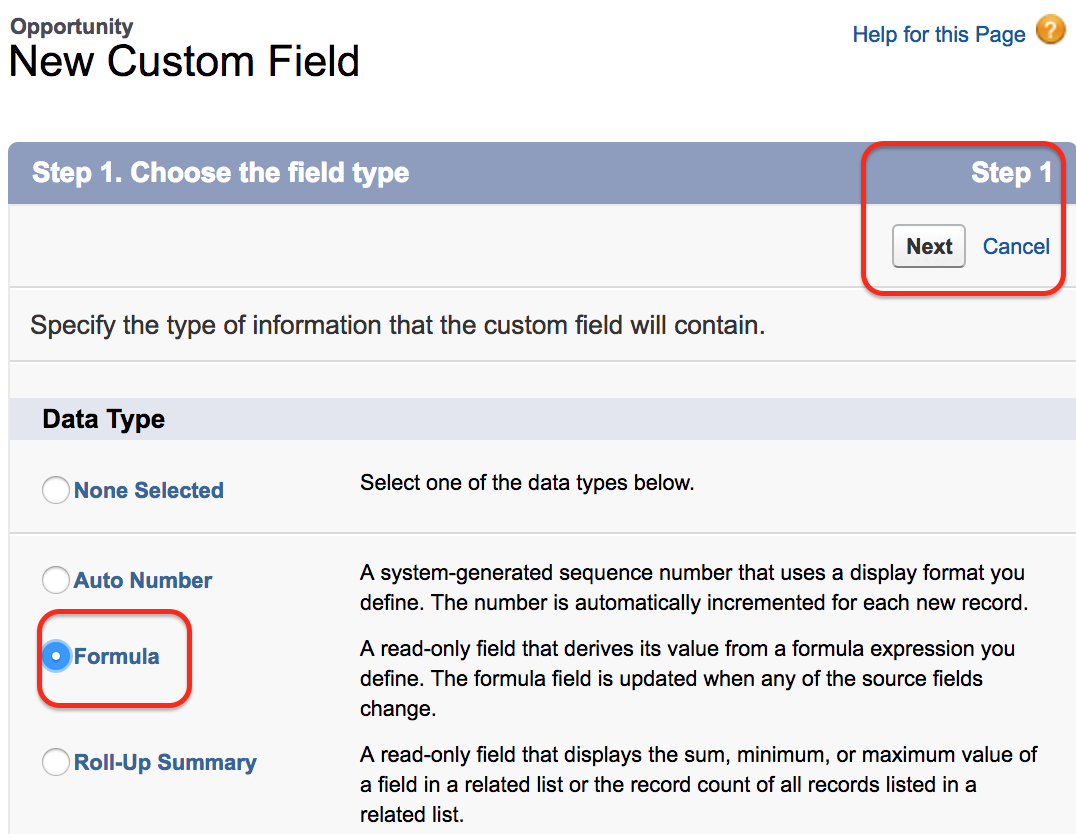In step 1 choose the field type as formula and click on Next button.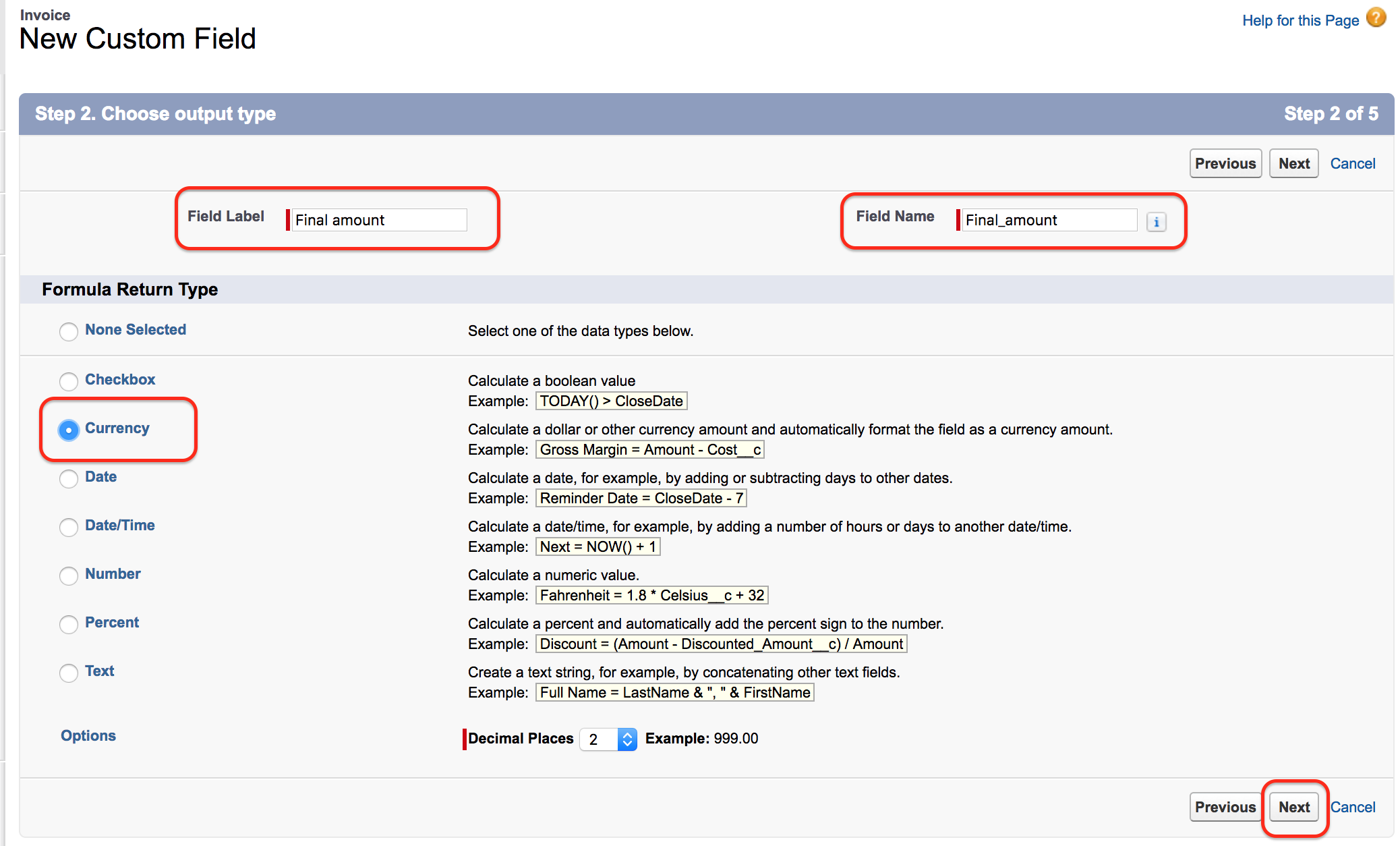Now select CurrencyField label  Field name  and click on next button and Enter formula field as shown in above Formula editor as shown above. Finally save all the settings as shown in our previous salesforce tutorials.

Let us check the working of Formulae Field.

• Now go to Invoice Object and create a new record in Invoice.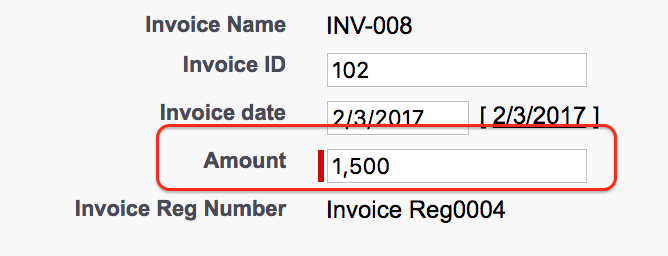• As shown above we have given amount as 1500\$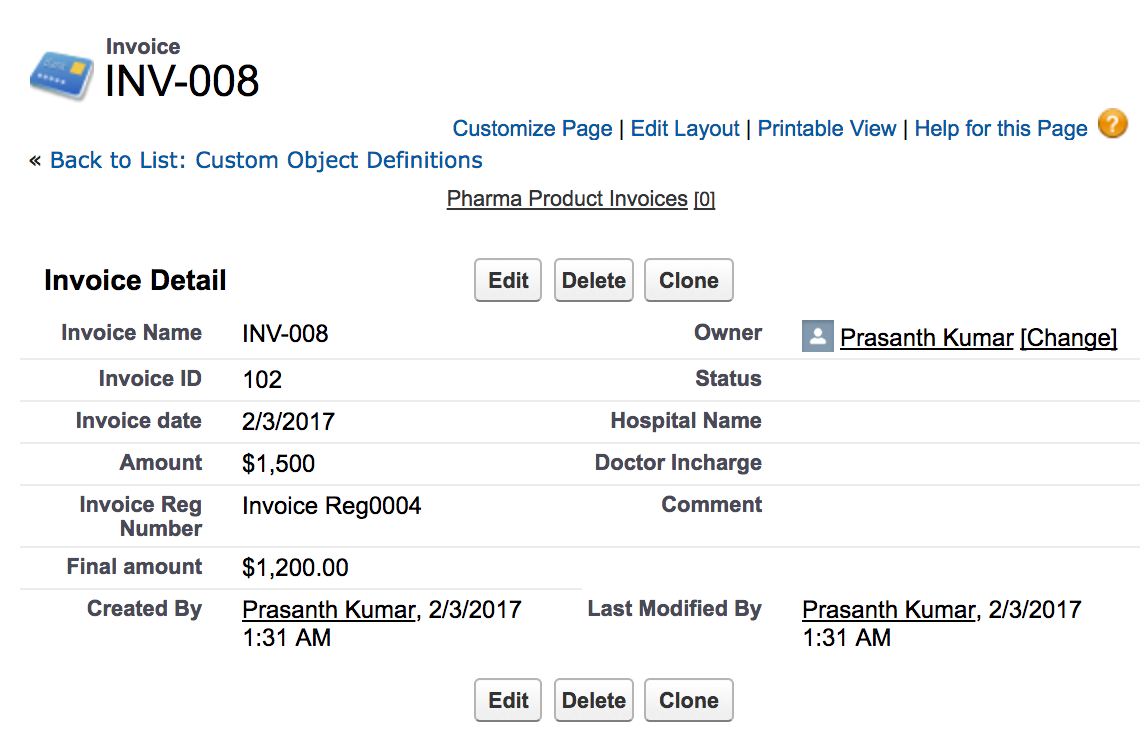After adding formula field we get the final amount as 1200\$.

Conclusion.

In this Salesforce tutorial we have learned about how to create a formula field in salesforce and about Formula editor. In our next Salesforce Tutorial we are going to learn about cross Object Formulas.